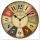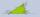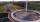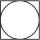# Subtracting - 6th grade (11y) - math problems

#### Number of examples found: 115

• MinutesDetermine the difference in minutes: T1 = 2 3/20 h T2 = 2.3 h
• Brackets 2Add parenthesis to make true: 5-2×6-4+2=5
• Pounds3 pounds subtract 1/3 of a pound.
• Expression 8Evaluate this expressions: a) 5[3 + 4(2.8 - 3)] b) 5×(8-4)÷4-2
• The temperature 2The temperature of Siberia fell13 degrees below 2/3 degrees centigrad. What is the temperature there now?
• Temperature change 3At 2 p. M. The temperature was 76 degrees Fahrenheit. At 8 p. M. The temperature was 58 degrees Fahrenheit. What was the change in temperature?
• Temperature changeThe mean temperature change is -3.2°F per day for five days. What is the total change over the five day period?
• Temperature 13Work out the temperature after each change. The temperature starts at 6°C and falls by 13°C. The temperature starts at 2°C and falls by 8°C
• Product of the sum and differenceCalculate the product of the sum and difference of numbers -7 and -2.
• Angles in triangleCalculate the alpha angle in the triangle if beta is 61 degrees and 98 gamma degrees.
• A number 2A number decreased by the difference between four and the number
• SchoolThere are 150 pupils in grade 5 . 2/3 of it are female. By what fractions are the males?
• Temperature differenceThe highest temperature of Libya was recorded 58 degree Celsius and the lowest was recorded -88 degree Celsius, what is the temperature difference?
• Expression 6Evaluate expression: -6-2(4-8)-9
• The rodThe rod has a length of 90cm. Half is painted blue, the third is red and the rest is yellow. How long is the yellow part of the rod?
• AnnulusCalculate the area of two circles annulus k1 (S, 3 cm) and k2 (S, 5 cm).
• Temperature variationsToday's temperature was 80 degrees, and then the temperature dropped 10 degrees. Then it dropped 15 degrees again, then the next day, the temperature went up 2 degrees. What would the temperature be?
• The lowestThe lowest temperature on Thursday was 20°C. The lowest temperature on Saturday was 12°C. What was the difference between the lowest temperatures?
• Unknown xIf we add to unknown number 21, then divide by 6 and then subtract 51, we get back an unknown number. What is this unknown number?
• Square and circleInto square is inscribed circle with diameter 10 cm.What is difference between circumference square and circle?

Do you have an interesting mathematical word problem that you can't solve it? Submit a math problem, and we can try to solve it.

We will send a solution to your e-mail address. Solved examples are also published here. Please enter the e-mail correctly and check whether you don't have a full mailbox.

Please do not submit problems from current active competitions such as Mathematical Olympiad, correspondence seminars etc...